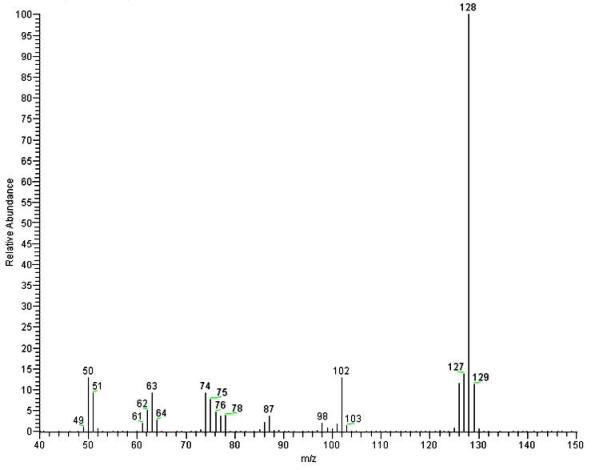# What is Mass Spectrometry? Share this page:Mass spectrometry involves the measurement of the mass-to-charge ratio of ions. Fundamental components of mass spectrometers include a means of sample introduction, a method of generating gas-phase ions, a mass analyzer (with a vacuum system), and an ion detector. Modern mass spectrometers have a data system for instrument control and data output.

A mass spectrum is the graph of ion intensity (or relative abundance) vs. mass (or, more properly, m/z - the mass-to-charge ratio).A useful overview of mass spectrometry may be found at en.wikipedia.org/wiki/Mass_spectrometry.

X